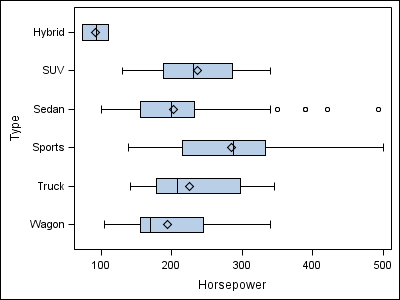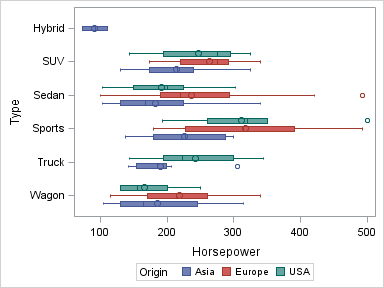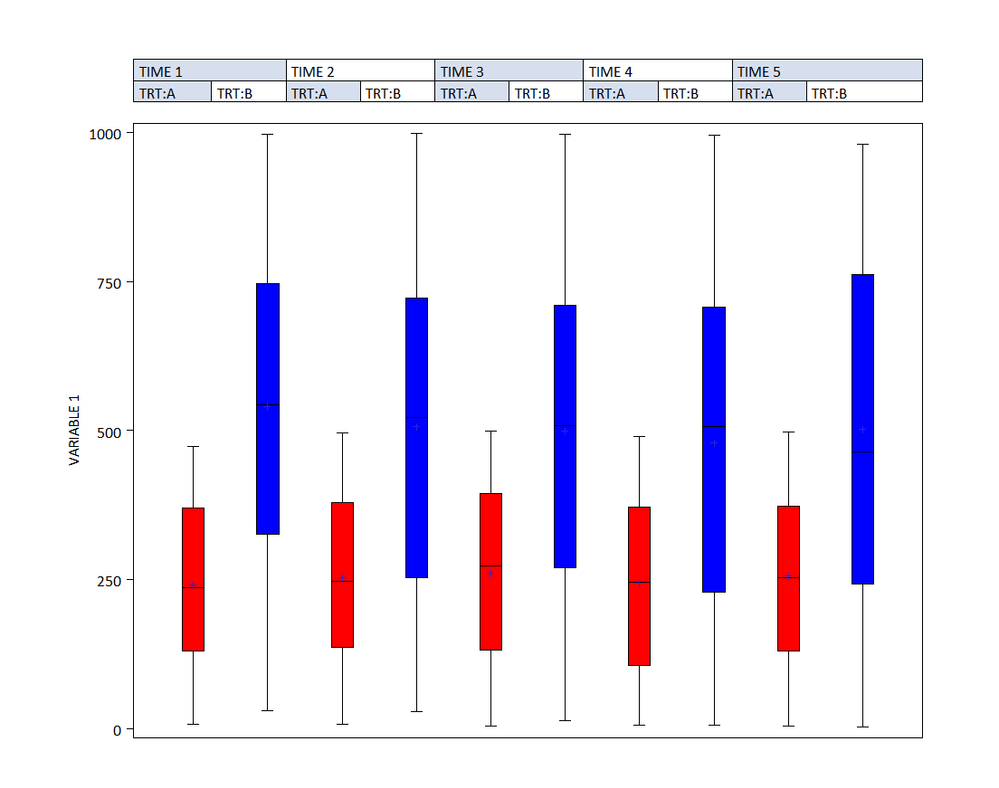## Alternating boxplot colors

Hello, I am programming boxplots using proc boxplot with a grouping variable (trt)

The code goes like this:

proc boxplot data = test3 ;

symbol value=dot height=1 c=blue;

plot var1* time (trt) / cframe   = vligb

cboxes   = dagr

cboxfill =ywh ;

My question is whether there is a way to fill the boxes with aternative colors - since I am comparing 2 treatment groups?  The x-axis is arranged such that for for time=1 I have 2 boxes above - one for trt A and one for trt B.  After that there is time=2 with 2 boxes above - A and B.  I want to differentiate between A and B.

5 REPLIES 5

## Re: Alternating boxplot colors

If none of the built-in boxplots (proc boxplot, gtl, or gplot with interpol=box) support this, you might have to use some custom programming to create it.

Here's an example I wrote, that draws basic boxplot geometry with annotate - it would require quite a bit of further customizing to get the exact boxplot you're wanting.  You could programmatically control the color of each box (based on values in the data), etc.  I guess the 'tough' part would be visually/spatially "grouping" the bars together, since the annotate coordinates are based on gplot, and gplot doesn't have support for official groups.

Here's the code:

I describe how the code works, in detail, in my book "SAS/Graph: Beyond the Basics" - it's Example 10, on pp. 65-70.

## Re: Alternating boxplot colors

Thanks Rob.

Your code looks quite complicated. It will take me some time.  I was hoping for a more "built in" solution. Is there a way to use annotate (with which I'm really not very familiar ) to color every other box? And can I use annotate with proc boxplot?Jay54
Meteorite | Level 14

## Re: Alternating boxplot colors

The answer depends on which release you are using.

At SAS 9.2 - A simple (non-grouped) Horizontal box plot can be made as below:

proc sgplot data=sashelp.cars;

hbox horsepower / category=type;

run;At SAS 9.3 - A grouped Horizontal box plot can be made as below:

proc sgplot data=sashelp.cars;

hbox horsepower / category=type group=origin groupdisplay=cluster;

run;But, you may not have SAS 9.3, in which case you can use SAS 9.2 GTL by overlaying two box plots, and use DiscreteOffsets to position them side by side.  See this article on Distribution of Max LFT.MikeZdeb
Rhodochrosite | Level 12

## Re: Alternating boxplot colors

hi ... here's a 'non-SG solution' ...

I made up some data similar to yours (sort of, maybe you can tweak your data a little to match what I used)

I used the CBOXFILL=(variable) option to change colors according to treatment ...

data x;

array color(2) \$4 _temporary_ ('red' 'blue');

do time=1 to 5;

do trt=1 to 2;

* vary color by treatment;

c=color(trt);

n+1;

* 100 observations at each time/treatment combo;

do k=1 to 100;

var1 = ceil(trt*500*ranuni(0));

output;

end;

end;

end;

drop k;

run;

proc sort data=x;

by n;

run;

proc format;

value trt

1='TRT:A' 2='TRT:B';

value tm

1='TIME 1' 2='TIME 2' 3='TIME 3' 4='TIME 4' 5='TIME 5';

run;

goptions reset=all gunit=pct ftext='calibri' htext=2;

axis1 value=none label=none major=none ;

axis2 label=('VARIABLE 1');

* white space around plot;

title1 ls=2;

title2 a=90 ls=2;

title3 a=-90 ls=2;

footnote1 ls=2;

proc boxplot data=x;

plot var1*n(trt time) / cboxfill=(c) haxis=axis1 vaxis=axis2;

format trt trt. time tm.;

run;

quit;## Re: Alternating boxplot colors

Thank you everyone for your help.

I got the box plots to alternate colors by assigning the colors based on the treatment and then using the cboxfill=(color variable name) as described above.

Discussion stats
• 5 replies
• 9513 views
• 1 like
• 4 in conversation